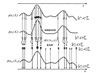Particle Filter

PF

• 粒子滤波及matlab实现 粒子滤波就是指通过寻找一组在状态空间中传播的随机样本来近似的表示概率密度函数用样本均值代替积分运算进而获得系统状态的最小方差估计的过程这些样本被形象的称为粒子故而叫粒子滤波粒子...
• Matlab基本的粒子滤波程序-粒子滤波程序.rar 粒子滤波程序.rar 相信对初学者有点帮助！！matlab
• 粒子滤波的原理和MATLAB实现
//预警，学得粗浅，语无伦次也不够完整，仅记录自己的理解。若有错误请不吝赐教
我师兄实验的全流程是（粒子滤波只是其中一环节）

拍摄一系列图片

对第一张图片用图像处理找出特征点
2.1. 彩色->灰度；中值滤波；阈值分割；二值化；
2.2. 形态学处理（加粗 填平）；面积域滤波
2.3. 小波边缘检测 ；提取中心线
2.4. 依据数据的特点找出特征点，记录特征点坐标值

以第一帧的特征点位置为参考 初始化之后遍历后续的图片获取特征点在这一系列图片中的坐标变化
3.1. 直接赋值初始化第一个特征点的图像位置
3.2. 以特征点为基准画一个框【ROI窗口】
3.3. STC跟踪算法函数读取下一张图片之后就能跟踪出新坐标，保存坐标值

这一系列坐标值就是观测值，要粒子滤波了【毕竟刚学，我很有可能搞错】
4.1. 赋值初始化第一个数据，并填入状态方程，加上噪声求得状态值，将求得的状态值加上噪声求得预测观测值
4.2. 开始对后续图像进行挨个操作
4.2. 1. 将上面求的状态值套入运动方程求得预测观测值【这就是为啥输入状态是k-1而噪声是k】
4.2. 2. 按高斯曲线给定权重 将预测观测值与测量值相减 取绝对值 偏差越小权值越大；然后权值归一化
4.2. 3. 重采样 把权值过小的值筛除，并用权值大的补上
4.2. 4 求状态值的平均值
4.3. 可以输出3条线。原始数据，滤波后，理想直线【可以用拟合，也可以直接定个直线】

输出各曲线与理想值的偏差、平均偏差、最大偏差。

下面开始知识记录
蒙特卡洛 //粒子滤波的前身
有一个求定积分的例子我印象深刻
随机投点法
a)首先，在x=[0,1]时，max（exp(x))=e，所以矩形面积为1*e；

b)然后，在矩形里面，均匀生成粒子（均匀分布生成的随机数，随机数范围为[Min(exp(x)),Max(exp(x))]），对于某个具体的粒子x，当x<exp(x)时，标记一次，做好记录，循环N次，看记录之和sum，然后得到概率P=sum/N

c)最后，用P*矩形面积=所求的面积.

贝叶斯滤波
按照前面1：k-1时刻的测量数据，预测一下状态x(k)出现的概率【先验概率密度】/因为会有噪声，所以即使状态方程是准的，从k-1状态预测k时也照样预测不准/。然后读取最新测量值对先验概率进行修正【后验概率密度】
粒子滤波
已知上一刻的状态值，求出这一刻的状态值1，再从传感器那边求出这一刻的状态值2，把这俩状态值相减，取绝对值，最新的数据权重最大 旧数据权重比较小但也算参考到了 ，而且之后要重采样 那俩状态值差值越小的越重要 相差太大的忽略不计。

k表示时刻，每次for循环k
状态方程 ： 某一时刻的状态矩阵 + 噪声矩阵， 输出预测状态值X
测量方程 ： 传感器实际测量值 + 传感器的噪声， 输出测量状态值Y//这些符号没有统一规定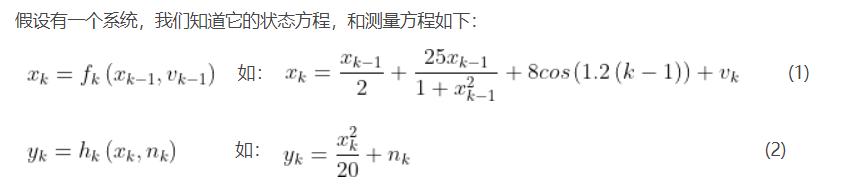上图状态方程里的Vk-1应改成Vk才是对的，而且后面举例写的也是Vk。

建立系统模型
假设匀速直线运动
目标状态是
$\Gamma X(k)=[p(k) , v(k) ]^{T}$
//那个自动冒出来的奇奇怪怪的符号是啥
位置 p(k+1)=p(k)+v(k)T+(1/2)aT²
速度 v(k+1)=v(k)+aT
矩阵形式就是
$\begin{gathered} \begin{bmatrix} p(k+1) \\ v(k+1) \end{bmatrix}= \begin{bmatrix} 1 & T \\ 0 & 1 \end{bmatrix} \begin{bmatrix} p(k) \\ v(k) \end{bmatrix}+ \begin{bmatrix} 0.5T² & 0 \\ 0 & T \end{bmatrix} \begin{bmatrix} a(k) \\ a(k) \end{bmatrix} \end{gathered}$
书里不知道为什么把位移的a和速度的a区分为两个a，有不同下标

在滤波中蒙特卡洛又是怎么用的呢？
由上面我们知道，它可以用来估计概率，而在上一节中，贝叶斯后验概率的计算里要用到积分，为了解决这个积分难的问题，可以用蒙特卡洛采样来代替计算后验概率。
假设可以从后验概率中采样到N个样本，那么后验概率的计算可表示为： 其中，在这个蒙特卡洛方法中，我们定义,是狄拉克函数(dirac delta function)，跟上面的指示函数意思差不多。
看到这里，既然用蒙特卡洛方法能够用来直接估计后验概率，现在估计出了后验概率，那到底怎么用来做图像跟踪或者滤波呢？要做图像跟踪或者滤波，其实就是想知道当前状态的期望值：
也就是用这些采样的粒子的状态值直接平均就得到了期望值，也就是滤波后的值，这里的 f(x)
就是每个粒子的状态函数。这就是粒子滤波了，只要从后验概率中采样很多粒子，用它们的状态求平均就得到了滤波结果。
思路看似简单，但是要命的是，后验概率不知道啊，怎么从后验概率分布中采样！所以这样直接去应用是行不通的，这时候得引入重要性采样这个方法来解决这个问题。

参考文献
《粒子滤波原理及应用 ——MATLAB仿真 》 黄小平 王岩  ；电子工业出版社；
蒙特卡洛方法-多重采样
粒子滤波（Particle filter）算法简介及MATLAB实现
particle filtering—粒子滤波（讲的很通俗易懂）
% 基于SIR算法的基本粒子滤波
% 准备仿真数据
%linspace(x1,x2,N)用于产生x1,x2之间的N点行矢量。其中x1、x2、N分别为起始值、终止值、元素个数
virtual_data=linspace(0,10,250)’+sqrt(0.04)*randn(250,1);%x方向偏移量10，分成250帧，加上随机噪声
Z=virtual_data;
% 运动模型
% x(t)=x(t-1)+10/250+w(t);
% y(t)=x(t)+v(t);
% w(t)~N(0,Q) 系统过程噪声为零均值，假设为高斯白噪声
% v(t)~N(0,R) 观测噪声假设为零均值，高斯白噪声
Q=0.001;
R=0.001;
T=250;  % 滤波回合数为250
particles_num=100;   % 粒子数为100
XParticleFilterOut=zeros(T,1);
XParticles=zeros(particles_num,T);   % 粒子
weight=zeros(particles_num,T);    % 粒子权重
Zpre_pf=zeros(particles_num,T);     % 粒子滤波观测预测值
Xpf=zeros(particles_num,T);     % 粒子滤波估计状态
% 给定状态的初始化采样
x0=virtual_data(1);%第一个仿真数据
Xpf(:,1)=x0+sqrt(Q)*rand(particles_num,1);%状态方程
Zpre_pf(:,1)=Xpf(:,1)+sqrt®*rand(particles_num,1); % 粒子滤波观测预测值 之后要用来和观测值相减
% 更新和预测过程
for k=2:T
% 第一步：粒子集合采样过程
for i=1:particles_num
QQ=Q;
net=sqrt(QQ)randn;%这里的QQ可以看成net的半径，可以改数值
XParticles(i,k)=Xpf(i,k-1)+ 10/250+ net;%对第一个仿真值之后的状态值按噪声进行调整
end
% 第二步：对粒子集合中的每个粒子，计算其重要性权值
for i=1:particles_num
Zpre_pf(i,k)=XParticles(i,k);
weight(i,k)=exp(-0.5R(-1)*(Z(k,1)-Zpre_pf(i,k))2);%用高斯函数确定权值 偏差越小权值越大
end
weight(:,k)=weight(:,k)./sum(weight(:,k)); % 归一化
% 第三步：重采样
for i=1:particles_num
outIndex=find(rand <= cumsum(weight(:,k)),1);
if length(outIndex) ~= 0
Xpf(:,k)=XParticles(outIndex,k);%把权值过小的值筛除，并用权值大的补上
end
end
XParticleFilterOut(k,1)=mean(Xpf(:,k));%求状态值的平均值
end
% 制图
ideal=linspace(0,10,250);
figure();
plot(Z,‘b’);
hold on;
plot(XParticleFilterOut,‘r’);
plot(ideal,‘k’);
%WQ_start
Filter_err=abs(XParticleFilterOut-ideal’);
Z_err=abs(Z-ideal’);
Filter_meanerr=mean(Filter_err);
Z_meanerr=mean(Z_err);
Filter_maxerr=max(Filter_err);
Z_maxerr=max(Z_err);
%WQ_end


展开全文• 基于当前统计模型的改进粒子滤波算法经典-基于当前统计模型的改进粒子滤波算法.rar 基于当前统计模型的改进粒子滤波算法matlab
• 粒子滤波初探 对于线性、高斯分布的运动模型，有卡尔曼滤波，那么对于非线性、非高斯的运动模型，粒子滤波器发挥出其优越性，主体思想是通过大量试验，对正确的试验状态予以适当的权重分配，从而实现根据权重增加的...
粒子滤波初探

对于线性、高斯分布的运动模型，有卡尔曼滤波，那么对于非线性、非高斯的运动模型，粒子滤波器发挥出其优越性，主体思想是通过大量试验，对正确的试验状态予以适当的权重分配，从而实现根据权重增加的方向实现跟踪。

本文与其他描述粒子滤波的博文不同，先以例子引入，让初步了解的人对粒子滤波有一个大致认识。

以视频的目标跟踪为例，解说一下这个粒子滤波跟踪流程。

一、t-1时刻下：假定我们有这么一个视频二、我们来初始化这个粒子滤波器

使上面的屏幕布满粒子
初始化每个粒子的权重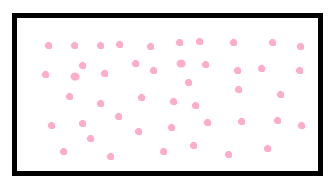三、如果视频中有个人，而且被我们框定作为目标

在利用重要性采样计算粒子权重之前，要记住这时候的粒子还是布满整个屏幕的，而且权重一样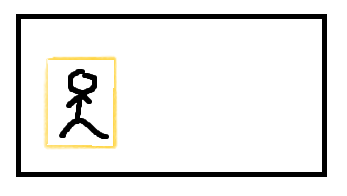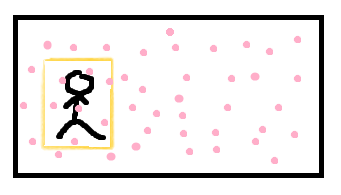四、更新权重

这时候，由于我们有了目标，就可以去计算粒子的权重了（我们暂时不去了解如何计算新的权重，反正粒子附近的图像与我们框定的图像内容越相似，其得到的更新权重就越高【想要了解的可看下一篇《粒子滤波初探（二）利用粒子滤波实现视频目标跟踪工程实战》的步骤四】）
下图中，颜色越鲜艳的代表该粒子权重越大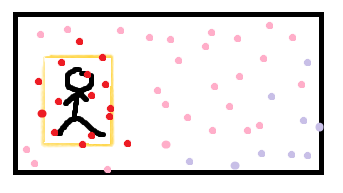五、重采样

接下来，很自然的想到，舍弃权重较小的粒子，但是为了维持粒子数不变，自然是要增加一些粒子，这就是重采样
于是有了下图
粒子群集中到我们框定的目标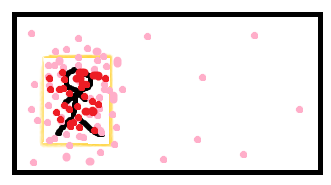六、然后，根据现在的权重分配及给定的状态转移矩阵，预测（生成）出下一时刻即t时刻粒子群的位置

假定预测的结果是这个样子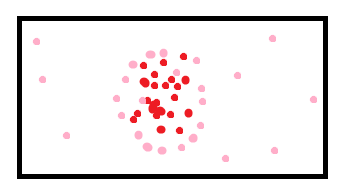以上都是t-1时刻下操作

七、进入t时刻（我以视频边框*2表示）

假定我们的人已经走到这个位置
下面4张图，上面两张是t-1时刻下的，下面两张是t时刻的
左下是我们的行人移动的位置
右下是我们的行人和t-1时刻预测出来的t时刻的粒子位置
从右下图可见，我们的预测貌似偏了一些，没关系，下一步将重新计算粒子的权重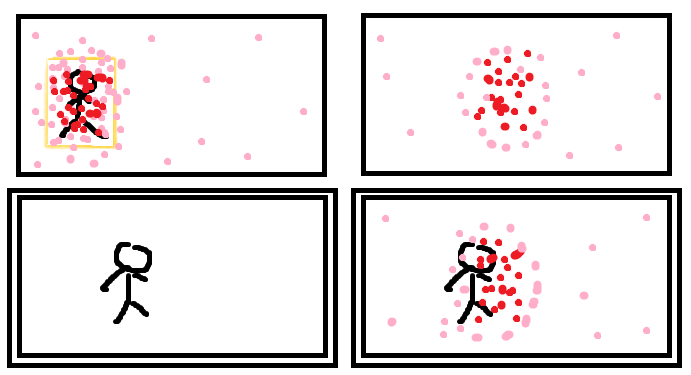八、更新权重

跟第四步一样，我们以t-1时刻下我们手动标注的橙色框为目标，重新更新我们粒子的权重
粒子还是那些粒子，只不过权重发生变化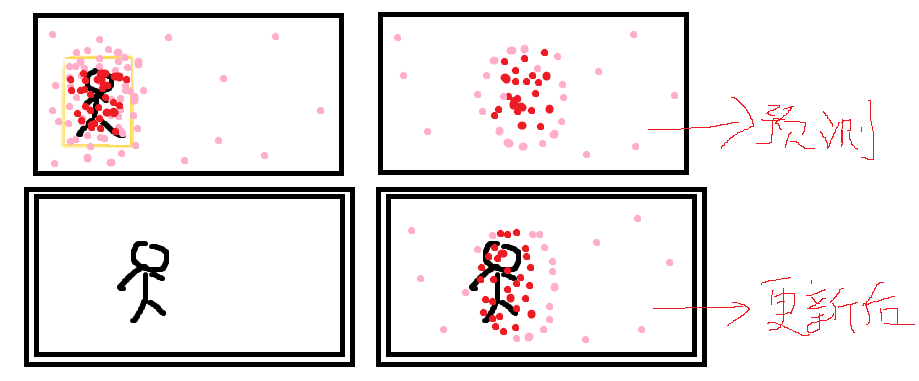九、重采样&&提取位置

重采样：去除权重低的粒子，复制权重高的粒子
根据重采样后的粒子分布，可以获取大致的目标位置，也就是我们跟踪的成果，如右下图蓝框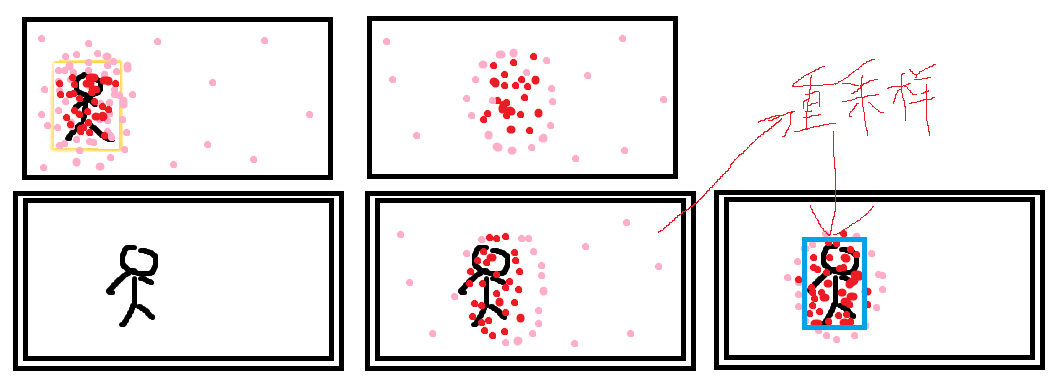十、更新目标

这里已经是t时刻的末端：
我们以此刻蓝框的内容作为我们的目标，以便t+1时刻用以更新粒子权重
我们以粒子群从t-1时刻到t时刻重采样后（即从橙色框移动到蓝色框的运动方向），作为粒子群下一次运动方向的依据，预测（生成）出t+1时刻粒子群的位置，接下来的步骤同第六步
到这里，t时刻结束，进入t+1时刻
到这里，一共十步，大致把这个粒子滤波的目标跟踪流程理了一遍。

下一篇：粒子滤波初探（二）利用粒子滤波实现视频目标跟踪工程实战 ，有可运行代码，是个人根据几位先驱做作出的努力翻译到opencv2.49下的，并做出了一些修正，希望各位指出不足和作出修正

参考资料：

https://blog.csdn.net/guoyunlei/article/details/78183530

https://blog.csdn.net/gwplovekimi/article/details/80359512

https://blog.csdn.net/yang_xian521/article/details/6928131

http://www.cnblogs.com/yangyangcv/archive/2010/05/23/1742263.html
展开全文...